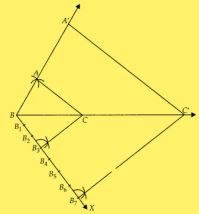# Chapter 10 Construction NCERT Exemplar Solutions Exercise 10.2 Class 10 MathsChapter Name NCERT Maths Exemplar Solutions for Chapter 10 Construction Exercise 10.2 Book Name NCERT Exemplar for Class 10 Maths Other Exercises Exercise 10.1Exercise 10.3Exercise 10.4 Related Study NCERT Solutions for Class 10 Maths

### Exercise 10.1 Solutions

Write ‘True’ or ‘False’ and justify your answer in each of the following:

1. By geometrical construction, it is possible to divide a line segment in the ratio √3 : 1/√3.

Solution

True
Explanation:
As given in the question
Ratio = √3 : 1/√3.
On solving,
√3 : 1/√3. = 3 : 1
Therefore, geometrical construction is possible to divide a line segment in the ratio 3 : 1.

2. To construct a triangle similar to a given △ABC with its sides 7/3 of the corresponding sides of △ABC, draw a ray BX making acute angle with BC and X lies on the opposite side of A with respect to BC. The points B1 , B2 , ……, B7 are located at equal distance on BX, B3 is joined to C and then a line segment B6C'  is drawn parallel to B3C where C' lies on BC produced.  Finally, line segment A'C' is drawn parallel to  AC.

Solution

False
Steps of construction:

1. Draw a line segment BC with suitable length.
2. Taking B and C as centers draw two arcs of suitable radii intersecting each other at A.
3. Join BA and CA. ∆ABC is the required triangle.
4. From B draw any ray BX downwards making an acute angle CBX.
5. Locate seven points B1 , B2 , B3 , .... B7  on BX such that BB1 = B1B2 = B1B3 = B3B4 = B4B1 = B5B6  = B6B7
6. Join B3C and from B7 draw a line B7C’ ॥ B3C intersecting the extended line segment BC at C’.
7. From point C’ draw C’A’ ॥ CA intersecting the extended line segment BA at A’.Then
∆A’BC’ is the required triangle whose sides are 7/3 of the corresponding sides of ∆ABC.
Given,
Segment B6C’ is drawn parallel to B3C.
But from our construction is never possible that segment B6C’ is parallel to B3C because the similar triangle A’BC’ has its sides 7/3 of the corresponding sides of triangle ABC.
Therefore, B7C’ is parallel to B3C.

3. A pair of tangents can be constructed from a point P to a circle of radius 3.5 cm situated at a distance of 3 cm from the centre.

Solution

False
As, the radius of the circle is 3.5 cm
r = 3.5 cm and a point P situated at a distance of 3 cm from the centre
So,
d = 3 cm.
We can see that r > d
Therefore, a point P lies inside the circle.
And, no tangent can be drawn to a circle from a point lying inside it.

4. A pair of tangents can be constructed to a circle inclined at an angle of 170°.

Solution

True
As, the angle between the pair of tangents is always greater than 0 but less than 180°.
Therefore, we can draw a pair of tangents to a circle inclined at an angle at 170°.